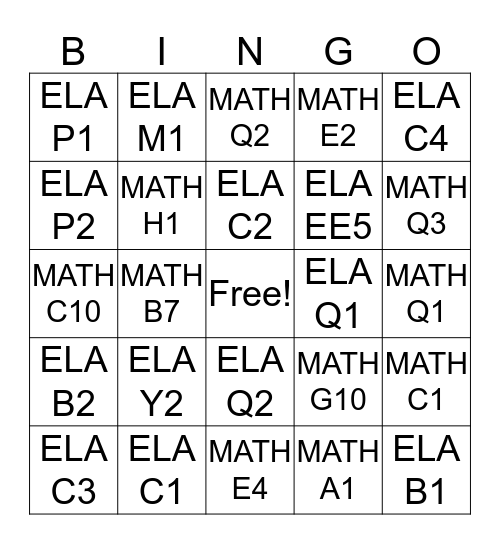# IXL 1This bingo card has a free space and 24 words: ELA Y2, ELA C1, ELA C2, ELA C3, ELA C4, ELA P1, ELA P2, ELA M1, ELA Q1, ELA Q2, ELA EE5, MATH C10, MATH G10, MATH C1, MATH H1, MATH A1, MATH Q1, MATH Q2, MATH Q3, MATH B7, ELA B1, ELA B2, MATH E2 and MATH E4.

## Play Online